The Ultimate DirectX Tutorial
Lesson 5: Drawing a Triangle
Lesson Overview

This is the first part of the tutorial where we will actually get to draw something! In this lesson you will learn to draw a triangle on the screen. We will build this triangle by creating a series of vertices and having the hardware draw the result for us.

This takes a lot of code. I won't pretend that rendering a triangle is as easy as "hello world", but it certainly will make sense in the end, and things will get easier as we go on. In the meantime, let's dive right in.

Rendering a triangle requires a number of actions to take place. This lesson is long, but is broken down into these parts:

1. First, we create three vertices to make a triangle.
2. Second, we store these vertices in video memory.
3. Third, we tell the GPU how to read these vertices.
4. Fourth, we tell the GPU how to translate those vertices into a flat image.
5. Fifth, we tell the GPU where on the backbuffer we want the image to appear.
6. Sixth, we finally render the triangle.

Whew! The good news is that by themselves, each of these steps is easy. If we take them up one at a time, this lesson should be over in a jiffy!

Creating A Triangle

If you went through Lesson 1 in any great detail, you will recall the definition of vertex: the location and properties of an exact point in 3D space. The location simply consists of three numerical values which represent the vertex's coordinates. The properties of the vertex are also defined using numerical values.

Direct3D uses what is called an input layout. An input layout is the layout of the data containing the location and properties of a vertex. It would be a format of data that you can modify and set according to your needs. Let's take a look at how this works exactly.

A vertex is made of a struct, which contains the data pertinent to creating whatever 3D image it is made for. To display the image, we will copy all the information to the GPU and then order Direct3D to render the data to the back buffer. However, what if we were forced to send all the data that could possibly be wanted for a vertex? This would happen.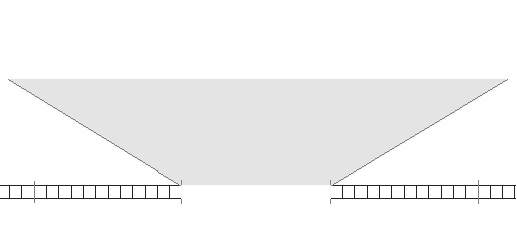A Vertex Format Containing All Possible Data

Of course, you may not see right away what the problem is here, but let's say we only needed two of these blocks of information. We could send it to the GPU much faster by doing it like this: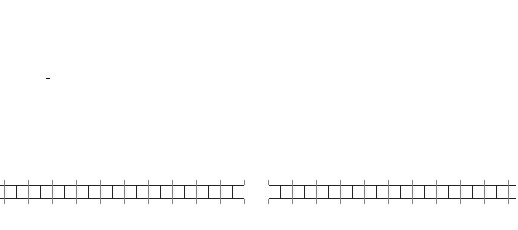A Selective Vertex Format Goes Faster

This is what happens when we use an input layout. We select which information we want to use, and send just that, enabling us to send many more vertices between each frame.

Creating Vertices

Let's start simple, and make a single vertex.

Vertices are typically created using a struct. In the struct you can place any data about the struct you like, in any format you like. For example, if we wanted each vertex to contain a position, we could build the following struct:

struct VERTEX
{
float X, Y, Z;    // vertex position
};

As you can see, we have three floats representing position along each axis.

Now let's build an actual vertex using this struct. We could do it like this:

VERTEX OurVertex = { 0.0f, 0.5f, 0.0f };

Of course, we could also make an array of vertices like this:

VERTEX OurVertices[] =
{
{ 0.0f, 0.5f, 0.0f },
{ 0.45f, -0.5f, 0.0f },
{ -0.45f, -0.5f, 0.0f },
};

This results in a triangle, which we will seen drawn on the screen shortly.

Let's create a new function in our CGame class for initializing geometry. I'll call it InitGraphics().

// this function loads and initializes all graphics data
void CGame::InitGraphics()
{

VERTEX OurVertices[] =
{
{ 0.0f, 0.5f, 0.0f },
{ 0.45f, -0.5f, 0.0f },
{ -0.45f, -0.5f, 0.0f },
};
}
Moving Geometry to Video Memory

When creating a stuct in C++ the data is stored in system memory. However, when the data is needed by the GPU, it's vital that the data be stored in video memory, which we don't have easy access to.

In order to allow us access to the video memory, Direct3D provides us with a specfic COM object that will let us maintain a buffer in both system and video memory. This object is called a vertex buffer.

How do buffers exist in both system and video memory? Well, initially the data in such a buffer will be stored in system memory. When rendering calls for it, Direct3D will automatically copy it over to video memory for you. If the video card becomes low on memory, Direct3D will delete buffers that haven't been used in a while, or are considered "low priority", in order to make room for newer resources.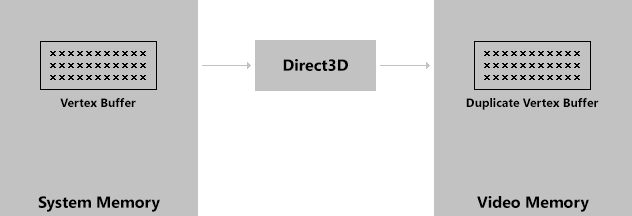Vertex Buffer in Video Memory When Needed

Why do we need Direct3D to do this for us? Well, it's very difficult to do this on your own, as accessing video memory varies depending on the video card and operating system version. Having Direct3D manage this for us is very, very handy.

This COM interface is called ID3D11Buffer. To create it, we use the CreateBuffer() function.

The CreateBuffer() function has three parameters. The first is a pointer to a struct describing the buffer. The second is a pointer to a struct describing the data to be stored (our triangle vertices). The third is a pointer to an ID3D11Buffer ComPtr.

Here's how you write the function out in practice:

ComPtr<ID3D11Buffer> vertexbuffer;    // defined in CGame

// this function loads and initializes all graphics data
void CGame::InitGraphics()
{
VERTEX OurVertices[] =
{
{ 0.0f, 0.5f, 0.0f },
{ 0.45f, -0.5f, 0.0f },
{ -0.45f, -0.5f, 0.0f },
};

D3D11_BUFFER_DESC bd = {0};
bd.ByteWidth = sizeof(VERTEX) * ARRAYSIZE(OurVertices);
bd.BindFlags = D3D11_BIND_VERTEX_BUFFER;

D3D11_SUBRESOURCE_DATA srd = {OurVertices, 0, 0};

dev->CreateBuffer(&bd, &srd, &vertexbuffer);

}

Here's what it all means.

D3D11_BUFFER_DESC bd = {0};

This is a struct containing properties of the buffer. Most members have 0 as their defaults, so we initialize the whole thing to 0.

bd.ByteWidth = sizeof(VERTEX) * ARRAYSIZE(OurVertices);

This value contains the size of the buffer that will be created. This is the same size as the array of vertices we intend to put into it. Considering the code we have so far, this value should be sizeof(VERTEX) * ARRAYSIZE(OurVertices).

bd.BindFlags = D3D11_BIND_VERTEX_BUFFER;

This value tells Direct3D what kind of buffer to make. There are several types of buffers we could make, but the kind we want to make is a vertex buffer. To do this, we can simply use the flag D3D11_BIND_VERTEX_BUFFER.

We won't go over the other flags here, as they are a bit advanced for this lesson. However, we will cover them all in due time.

D3D11_SUBRESOURCE_DATA srd = {OurVertices, 0, 0};

This is a struct containing a description of the data we wish to store in the vertex buffer. This is a short struct, so we can easily initialize it in one line.

The first value is a pointer to the data. This would be our array of vertices, so we just put OurVertices here.

The second and third values are advanced and we won't use them, so just set them to 0.

dev->CreateBuffer(&bd, &srd, &vertexbuffer);

The function that creates the buffer. As mentioned before, we include addresses to both structs and the ComPtr for our buffer.

This is a key part of 3D programming, and we'll be using it and modifying quite a bit as we go on. At this point I would suggest going over this section a couple times to make sure you really get it.

The process of rendering is controlled by what is known as the rendering pipeline. It is a series of steps which take vertices as input and result in a fully rendered image. Unfortunately, the pipeline does not automatically know what to do. It must first be programmed, and it is programmed by shaders.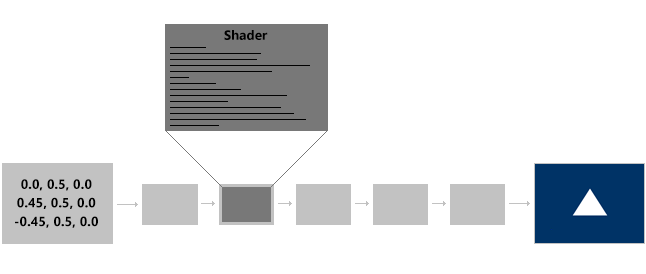There are several different types of shaders, and each is run many times during rendering. For example, a vertex shader is a program that is run once for each vertex rendered, while a pixel shader is a program that is run for each pixel drawn.

We will get to programming shaders in the next lesson. Right now, we are trying to render a triangle, and to do that we must load certain shaders to the GPU.

Each of these steps are very simple. Let's go over them quickly.

Burried in Visual Studio's New Item dialog is a menu titled HLSL. This stands for High Level Shader Language, and it's the language in which shaders are written. We'll learn about how to write HLSL over the next few lessons, but for now we want to create a few basic HLSL files.

Now, under the Visual C++ menu, select HLSL. There will be a list of seven files. You want to create a Vertex Shader file and a Pixel Shader file.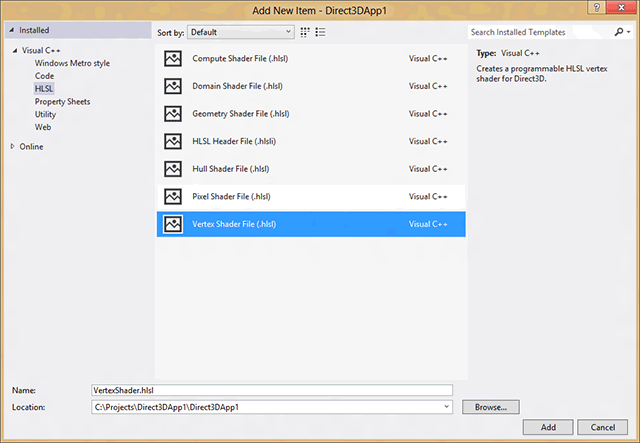The code in these files will not necessarily be familiar to you. Leave them be for now, and we'll learn to manipulate them soon.

When you compile your program, HLSL files automatically compile as well. However, they do not compile the way .cpp files compile. .hlsl files become .cso files, which stands for "Compiled Shader Object".

When our program runs, we must load the contents of each .cso file.

I've written a simple function that loads the contents of a file and stores it into an Array^. We can place this function at the top of Game.cpp.

#include <fstream>

// this function loads a file into an Array^
{
Array<byte>^ FileData = nullptr;

// open the file
std::ifstream VertexFile(File, std::ios::in | std::ios::binary | std::ios::ate);

// if open was successful
if(VertexFile.is_open())
{
// find the length of the file
int Length = (int)VertexFile.tellg();

// collect the file data
FileData = ref new Array<byte>(Length);
VertexFile.seekg(0, std::ios::beg);
VertexFile.close();
}

return FileData;
}

It's a tad over-simplified, but it gets the job done. It doesn't do anything to the data. It simply loads the file and dumps the contents into an Array^.

I'm going to write all the following code in a new function called InitPipeline() inside the CGame class. In this function we'll load shaders and do other prep work.

// this function initializes the GPU settings and prepares it for rendering
void CGame::InitPipeline()
{
}

To load the shaders, all we have to do is call the above LoadShaderFile() for each shader (.cso) file. Remember, .hlsl files become .cso files when you compile.

// this function initializes the GPU settings and prepares it for rendering
void CGame::InitPipeline()
{

}

Pretty simple.

A special COM object exists for every type of shader. For the vertex and pixel shaders, the interfaces to these objects are ID3D11VertexShader and ID3D11PixelShader.

// somewhere in the CGame class

Creating each of these objects is a single-function task. It's Create_____Shader(), where the blank is the type of shader. The parameters are a pointer to the shader file data, the size of the data, an advanced parameter we'll set to nullptr, and the address of the appropriate ComPtr.

// this function initializes the GPU settings and prepares it for rendering
void CGame::InitPipeline()
{

}

VSFile and PSFile have members called Data and Length, and they provide us with just that, the data and the length of the data.

This part is simple, so I'll just show the code and then explain it.

// this function initializes the GPU settings and prepares it for rendering
void CGame::InitPipeline()
{

}

The first parameter is the address of the shader object (using the Get() function), while the other two parameters are advanced and will be covered later.

And that's all we need to do with shaders for now. We've got quite a few lessons up ahead which will go into shaders in detail, so if you aren't quite following along, don't worry about it too much at this point.

Defining the Input Layout

So far in this lesson we have:

A) created a shape using vertices, and
B) loaded them into an object that moves them to video memory.

You may be wondering how the GPU is capable of reading our vertices, when we placed them in our own, self-created struct. How can it know that we included the Z position? How can it know that we didn't include other vertex properties?

The answer is the input layout.

As mentioned before, we are able to select what information gets stored with each vertex in order to improve the speed of rendering. The input layout is an object which contains the vertex struct's layout, letting the GPU organize the data appropriately and efficiently.

The ID3D11InputLayout object stores the layout of our VERTEX struct. To create this object we call the CreateInputLayout() function.

There are two parts to this. First, we need to define each element of the vertex. Second, we need to create the input layout object.

Creating the Input Elements

A vertex layout consists of one or more input elements. An input element is one property of the vertex, such as position, color, texture info, or something else.

Each element is defined by a struct, called D3D11_INPUT_ELEMENT_DESC.

D3D11_INPUT_ELEMENT_DESC ied = {0};
ied.SemanticName = "POSITION";
ied.Format = DXGI_FORMAT_R32G32B32_FLOAT;
ied.D3D11_INPUT_PER_VERTEX_DATA;

There are seven values in this struct, but we are only concerned with these three for now.

ied.SemanticName = "POSITION";

A semantic is a string which tells the GPU what the value is used for. There are numerous semantics possible, but we'll learn them as we come to them. "POSITION" represents a 3-dimensional position using float values.

ied.Format = DXGI_FORMAT_R32G32B32_FLOAT;

This value is represents format of the data. On many semantics, the number of values is arbitrary (so long as its less than four). All that matters is that the format matches what you use in your vertices.

The format here shows three float values: one for red, green, and blue. Yes, we aren't talking about color, but position is stored the same exact way, and there isn't a special value for X, Y, and Z.

ied.D3D11_INPUT_PER_VERTEX_DATA;

This value is what the element is used as. There are two possible flags here, but the one we are interested in is D3D11_INPUT_PER_VERTEX_DATA. We'll cover the other flag later.

Because vertices commonly have more than one property, it's normal to put this struct in an array, and intialize each struct all at once.

// this function initializes the GPU settings and prepares it for rendering
void CGame::InitPipeline()
{

// initialize input layout
D3D11_INPUT_ELEMENT_DESC ied[] =
{
{"POSITION", 0, DXGI_FORMAT_R32G32B32_FLOAT, 0, 0, D3D11_INPUT_PER_VERTEX_DATA, 0},
};
}

There are seven values here, but only these three are needed for now.

Multiple Input Elements

In most cases, you won't be using one input element, you'll be using at least two. There are a few things to know about using multiple input elements.

Let's say we wanted to send a vertex with two color as well as position. We could add a second element to our array, like this:

D3D11_INPUT_ELEMENT_DESC ied[] =
{
{"POSITION", 0, DXGI_FORMAT_R32G32B32_FLOAT, 0, 0, D3D11_INPUT_PER_VERTEX_DATA, 0},
{"COLOR",    0, DXGI_FORMAT_R32G32B32_FLOAT, 0, 0, D3D11_INPUT_PER_VERTEX_DATA, 0},
};

The would work great, but Direct3D needs a little bit more information. It needs to know how many bytes into your structure a specific element begins. In other words, the COLOR element does not start at the beginning, and we have to say where it starts.

To do this, we use the fifth value in the struct. We count the bytes leading up to it, then use that number. For example, a float takes up 4 bytes. We know POSITION has three floats, so COLOR should start on byte 12. The new code looks like this:

D3D11_INPUT_ELEMENT_DESC ied[] =
{
{"POSITION", 0, DXGI_FORMAT_R32G32B32_FLOAT, 0, 0,  D3D11_INPUT_PER_VERTEX_DATA, 0},
{"COLOR",    0, DXGI_FORMAT_R32G32B32_FLOAT, 0, 12, D3D11_INPUT_PER_VERTEX_DATA, 0},
};

Well that's unfortunately complicated. The good news is once you write this code for your game, you rarely have to rewrite it or change it.

Creating the Input Layout Object

Once we have an array of structs representing our input layout, we need to create yet another COM object that manages them for us. This one is called ID3D11InputLayout. The function to create one is CreateInputLayout().

CreateInputLayout is really simple:

// this function initializes the GPU settings and prepares it for rendering
void CGame::InitPipeline()
{

// initialize input layout
D3D11_INPUT_ELEMENT_DESC ied[] =
{
{"POSITION", 0, DXGI_FORMAT_R32G32B32_FLOAT, 0, 0, D3D11_INPUT_PER_VERTEX_DATA, 0},
};

// create the input layout
dev->CreateInputLayout(ied, ARRAYSIZE(ied), VSFile->Data, VSFile->Length, &inputlayout);

}

The first parameter is the address of the element description array. The second parameter is the number of elements in the array (1 in our case, but we'll still use ARRAYSIZE).

The second and third parameters are the data and length of the vertex shader file. When CreateInputLayout() is called, Direct3D will check our input layout against the vertex shader to make sure they match up correctly. If they don't the function will fail (don't worry, they do match).

The final parameter is the address of the input layout object.

Setting the Input Layout Object.

Just like shader objects and render targets, once we've created the input layout object, it needs to be set. The function to set an input layout is really, really complicated.

ComPtr<ID3D11InputLayout> inputlayout;    // the input layout interface

// this function initializes the GPU settings and prepares it for rendering
void CGame::InitPipeline()
{

// initialize input layout
D3D11_INPUT_ELEMENT_DESC ied[] =
{
{"POSITION", 0, DXGI_FORMAT_R32G32B32_FLOAT, 0, 0, D3D11_INPUT_PER_VERTEX_DATA, 0},
};

// create and set the input layout
dev->CreateInputLayout(ied, ARRAYSIZE(ied), VSFile->Data, VSFile->Length, &inputlayout);
devcon->IASetInputLayout(inputlayout.Get());
}

I know, right? So not complicated!

Setting the Viewport

This section is a piece of cake compared to the others.

A viewport is a way of telling Direct3D how to place normalized device coordinates. We learned about those back in lesson 1.

Under most circumstances, the normalized coordinates run from the top left of the screen to the bottom right of the screen. But in some cases, that may not be what you want.

Before we can draw anything, Direct3D needs us to clarify how we want normalized coordinates to be layed out.

What -1, -1 and 1, 1 actually equate to in pixels is determined by the viewport. The viewport is a struct that lets us set where -1, -1 and 1, 1 will be in pixel coordinates.

Here's what the code to set the viewport looks like:

// this function initializes and prepares Direct3D for use
void CGame::Initialize()
{
// Direct3D initialization
// ...

// create the swap chain
// ...

// set the render target
// ...

// set the viewport
D3D11_VIEWPORT viewport = {0};

viewport.TopLeftX = 0;
viewport.TopLeftY = 0;
viewport.Width = Window->Bounds.Width;
viewport.Height = Window->Bounds.Height;

}

This code isn't too complex. We use Bounds.Width and Bounds.Height from within the Window class to determine the width and height of the window.

The function RSSetViewports() could use a little explaining too. It's a function that activates viewport structs. The first parameter is the number of viewports being used, and the second parameter is the a list of pointers to the viewport structs.

Using multiple viewports is handy in certain advanced situations, but we won't get into them here. For now, 1 and '&viewport' will suit the parameters fine.

Drawing the Primitive

Now let's get down to business.

There are three simple functions that we have to call to perform rendering. The first one sets which vertex buffer we intend to use. The second sets which type of primitive we intend to use (such as triangle lists, line strips, etc.) The third actually draws the shape.

IASetVertexBuffers()

The first of these functions is IASetVertexBuffers(). This will tell the GPU which vertices to read from when rendering. It has a couple of easy parameters, so let's look at the protoype:

void IASetVertexBuffers(UINT StartSlot,
UINT NumBuffers,
ID3D11Buffer **ppVertexBuffers,
UINT *pStrides,
UINT *pOffsets);

The first parameter is advanced, so we'll set it to 0 for now.

The second parameter tells how many buffers we are setting. Because we only have one buffer, we'll put 1 here.

The third parameter is a pointer to an array of vertex buffers. We only have one, so we can fill this with vertexbuffer.GetAddressOf().

The fourth parameter points to an array of UINTs, which tell the sizes of a single vertex in each vertex buffer. To fill this parameter we create a UINT, fill it with "sizeof(VERTEX)", and put the address of that UINT here.

The fifth parameter is an array of UINTs telling the number of bytes into the vertex buffer we should start rendering from. This will usually be 0. To do this, we create a UINT of 0 and put the address here.

It's a fairly simple function. Here's what it looks like:

// this function renders a single frame of 3D graphics
void CGame::Render()
{
// clear the back buffer to a deep blue
float color = {0.0f, 0.2f, 0.4f, 1.0f};
devcon->ClearRenderTargetView(rendertarget.Get(), color);

// set the vertex buffer
UINT stride = sizeof(VERTEX);
UINT offset = 0;

// switch the back buffer and the front buffer
swapchain->Present(1, 0);
}

IASetPrimitiveTopology

This second function tells Direct3D which type of primitive that is used. These were covered in Lesson 1, but the codes used are here:

ValueMeaning
D3D11_PRIMITIVE_TOPOLOGY_POINTLISTShows a series of points, one for each vertex.
D3D11_PRIMITIVE_TOPOLOGY_LINELISTShows a series of separated lines.
D3D11_PRIMITIVE_TOPOLOGY_LINESTRIPShows a series of connected lines.
D3D11_PRIMITIVE_TOPOLOGY_TRIANGLELISTShows a series of separated triangles.
D3D11_PRIMITIVE_TOPOLOGY_TRIANGLESTRIPShows a series of connected triangles.

The only parameter of this function is one of these flags. It is written out like this:

// this function renders a single frame of 3D graphics
void CGame::Render()
{
// clear the back buffer to a deep blue
float color = {0.0f, 0.2f, 0.4f, 1.0f};
devcon->ClearRenderTargetView(rendertarget.Get(), color);

// set the vertex buffer
UINT stride = sizeof(VERTEX);
UINT offset = 0;

// set the primitive topology
devcon->IASetPrimitiveTopology(D3D11_PRIMITIVE_TOPOLOGY_TRIANGLELIST);

// switch the back buffer and the front buffer
swapchain->Present(1, 0);
}

Draw()

Now that we have told Direct3D what kind of primitives to render, and what vertex buffer to read from, we tell it to draw the contents of the vertex buffer.

This function draws the primitives in the vertex buffer to the back buffer. Here is the prototype:

void Draw(UINT VertexCount,             // the number of vertices to be drawn
UINT StartVertexLocation);    // the first vertex to be drawn

These parameters control which vertices in the buffer to draw. The second parameter is a number telling the first vertex in the buffer that should be drawn, while the first parameter is the number of vertices that should be drawn.

In practice, the function looks like this:

// this function renders a single frame of 3D graphics
void CGame::Render()
{
// clear the back buffer to a deep blue
float color = {0.0f, 0.2f, 0.4f, 1.0f};
devcon->ClearRenderTargetView(rendertarget.Get(), color);

// set the vertex buffer
UINT stride = sizeof(VERTEX);
UINT offset = 0;

// set the primitive topology
devcon->IASetPrimitiveTopology(D3D11_PRIMITIVE_TOPOLOGY_TRIANGLELIST);

// draw 3 vertices, starting from vertex 0
devcon->Draw(3, 0);

// switch the back buffer and the front buffer
swapchain->Present(1, 0);
}

A Quick Review

Because a lot of code was covered in this lesson, let's stop and take a quick review of the whole thing. We'll start at the beginning and walk through everything again.

Vertex Buffers

In this section we learned to create a struct representing a vertex, and to create a Vertex Buffer object that handled the vertices in video memory. We built a function called InitGraphics().

// a struct to represent a single vertex
struct VERTEX
{
float X, Y, Z;    // vertex position
};

ComPtr<ID3D11Buffer> vertexbuffer;    // defined in CGame

// this function loads and initializes all graphics data
void CGame::InitGraphics()
{
// create a triangle out of vertices
VERTEX OurVertices[] =
{
{ 0.0f, 0.5f, 0.0f },
{ 0.45f, -0.5f, 0.0f },
{ -0.45f, -0.5f, 0.0f },
};

// create the vertex buffer
D3D11_BUFFER_DESC bd = {0};
bd.ByteWidth = sizeof(VERTEX) * ARRAYSIZE(OurVertices);
bd.BindFlags = D3D11_BIND_VERTEX_BUFFER;

D3D11_SUBRESOURCE_DATA srd = {OurVertices, 0, 0};

dev->CreateBuffer(&bd, &srd, &vertexbuffer);
}

In this function we:

1. Created three vertices forming a triangle.
2. Created a vertex buffer object.
3. Filled that vertex buffer object with out three vertices.

Shaders are mini-programs which are used to direct the GPU in rendering. Rendering is impossible without shaders. In this lesson we loaded two shaders and prepared them for the GPU.

Then we covered how to coordinate the vertex buffer and the shader by using an input layout object.

We placed all of this into the InitPipeline() function, shown below:

// defined in CGame
ComPtr<ID3D11InputLayout> inputlayout;

// this function initializes the GPU settings and prepares it for rendering
void CGame::InitPipeline()
{

// initialize input layout
D3D11_INPUT_ELEMENT_DESC ied[] =
{
{"POSITION", 0, DXGI_FORMAT_R32G32B32_FLOAT, 0, 0, D3D11_INPUT_PER_VERTEX_DATA, 0},
};

// create and set the input layout
dev->CreateInputLayout(ied, ARRAYSIZE(ied), VSFile->Data, VSFile->Length, &inputlayout);
devcon->IASetInputLayout(inputlayout.Get());
}

Here's all we did:

2. Used the data from the file to create two shader objects.
4. Created an "array" of input element descriptions (with only one in it).
5. Created an input layout object using that.
6. Set the input layout object.

Viewports

The viewport specifies what part of the back buffer should be drawn on. In this lesson, all we needed to do was create a viewport struct and set it.

// this function initializes and prepares Direct3D for use
void CGame::Initialize()
{
// Direct3D initialization
// ...

// create the swap chain
// ...

// set the render target
// ...

// set the viewport
D3D11_VIEWPORT viewport = {0};

viewport.TopLeftX = 0;
viewport.TopLeftY = 0;
viewport.Width = Window->Bounds.Width;
viewport.Height = Window->Bounds.Height;

}

Drawing the Primitive

Finally we actually got to draw the triangle. We added a few lines of code to the RenderFrame() function.

// this function renders a single frame of 3D graphics
void CGame::Render()
{
// clear the back buffer to a deep blue
float color = {0.0f, 0.2f, 0.4f, 1.0f};
devcon->ClearRenderTargetView(rendertarget.Get(), color);

// set our new render target object as the active render target

// set the vertex buffer
UINT stride = sizeof(VERTEX);
UINT offset = 0;

// set the primitive topology
devcon->IASetPrimitiveTopology(D3D11_PRIMITIVE_TOPOLOGY_TRIANGLELIST);

// draw 3 vertices, starting from vertex 0
devcon->Draw(3, 0);

// switch the back buffer and the front buffer
swapchain->Present(1, 0);
}

In here we:

1. Set which vertex buffer to use (there was only one to choose from).
2. Set which primitive type to use.
3. Drew the triangle.

The Finished Program

When building this program, make sure to include the two shader files: "VertexShader.hlsl" and "PixelShader.hlsl". Create them using Visual Studio's New Item dialog, leave them untouched, and you should be fine.

Don't change the contents of those files yet. That's important.

Go ahead and update your program and let's see what we get. If you run this, you should see the following on your screen:The Rendered Triangle

Well, this lesson covered quite a big step. There are many little parts to it, not all of which may seem relevant. In order to get a better idea of what's happening, answer these questions and do these exercises. They'll get you ready for what's to come.

Questions

1. What is a shader and what does it do?
2. What is a vertex buffer?
3. How is a vertex buffer different from a simple array of vertices?
4. How do input layouts make vertex buffers more efficient?

Exercises

1. Change the shape of the triangle.
2. Try changing the values of the viewport and see what happens.
3. Render using lines or points.
4. Make two triangle appear instead of one.

Next Lesson: The Rendering Pipeline

GO! GO! GO!